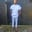Related Tags

laravel
php
communitycreator

# How to generate random numbers from a pool of numbers in LaravelChinweuba Elijah Azubuike

### Overview

In this shot, we will learn how to generate numbers from a pool with the random() method.

### What is the random() method?

The random() method is a Laravel array method that helps select random numbers from an array.

### Syntax

$random = Arr::random($arr,5);


### Parameters

The random() method takes two parameters:

1. An array that contains numbers.
2. The number of random numbers you want.

### Example

$arr = [9,10,11,12,13,14];$random = Arr::random($arr, 2);//randomly selecting 2 numbers from the numbers in the array return$random;



### Example explained

Don’t forget to import the Illuminate\Support\Arr; class.

$arr can be from your database, or you can manually set it like the example above. You then pass $arr to the random() method as the first parameter, as well as the number of random numbers you want as output. For the random() method to work, we have to call it on the Arr facade, which helps manipulate arrays.

### Output

While outputting more than one number, the random() method outputs an array.

For example, output=[3,4];.

RELATED TAGS

laravel
php
communitycreator

CONTRIBUTORChinweuba Elijah Azubuike
RELATED COURSES

View all Courses

Keep Exploring

Learn in-demand tech skills in half the time